### 5.2 The Fourier technique

While a complete presentation of Fourier analysis would require a full mathematical explanation and is hence beyond the scope of this chapter, there are a few aspects that are key to understanding the weave and angle maps that are produced using this technique. Fourier analysis is based on ‘sinusoids’ which may be pictured as oscillations of up to down, of high to low, or of dark to light. The oscillations are characterized by their frequency, which is how many cycles occur within a given image. For example, figure  shows several images with different numbers of horizontal and vertical frequencies. The basic idea behind Fourier analysis is to take an arbitrary signal (such as a region from a canvas X-ray) and to find the best match between the sinusoidal oscillations and the region.

The output of the Fourier transform is a picture called the spectrum, which plots the frequencies of oscillation (on the horizontal and vertical axes) against the quality of the match between each oscillation and the signal. When plotted in grayscale, a poor match is indicated in white and a good match in black. For example, suppose the image consists of 5 vertical and 2 horizontal oscillations as shown figure (a). The magnitude spectrum of this region consists of 4 black dots, shown in (b). Part (c) zooms in, and it becomes clear that the dark spots are located at +/-2 horizontal and +/-5 vertical from the center. Usually, the computer would be used to automatically select the dark spots and thus automatically identify the frequencies in the image. (d) shows an alternative way to visualize the transform in which the values of the spectrum are represented as height in a (pseudo) 3D landscape, rather than as darker and lighter blobs. This representation shows why the dark locations in (c) are often called ‘peaks’.

The spectrum is a picture that describes the number of oscillations in both the vertical and horizontal directions within the region of interest, at least for the simple sinusoidal model of figure 1(d) in § 5.1. The rectangular model from figure 1(c), § 5.1, also has a spectrum that has clear set of peaks that correspond to the density of the vertical and horizontal stripes, as shown in figure . Though the pattern of peaks is somewhat different than in figure 2, the distance in pixels from the origin/center to the dark spots along the axes are equal to the number of oscillations in each direction. Observe also that the rotation of the original image is preserved in the rotation of the spectrum. Again, the right hand image (c) shows the spectrum plotted as a topographic map; the locations of the peaks on the right are determined by the periodicities in the region on the left. In these images (and all spectra in this chapter), the average value of the image is removed to remove an uninteresting peak at the center point.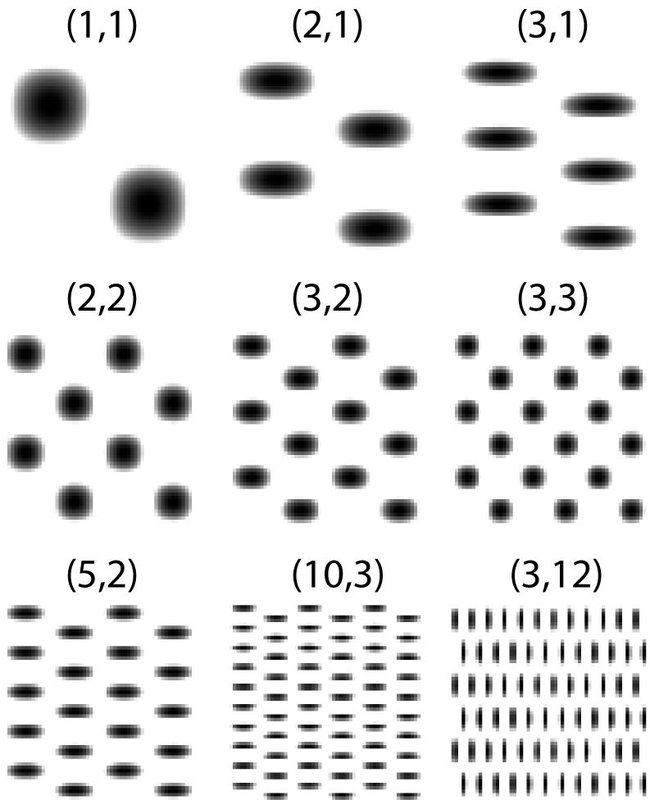1
Two-dimensional sinusoidal elements have both vertical and horizontal frequencies: a frequency of (5,2) means five vertical periods and two horizontal periods across the image, where each period consists of a smooth oscillation from dark to light and back to dark. Look down each column and there are five transitions from dark to light; look across any given row and there are two transitions from dark to light (or from light to dark in the staggered rows). The Fourier transform shows how a given image can be decomposed into a weighted sum of such sinusoidal functions, thus finding the best possible fit between the signal (in this case, a region of the X-ray image) and all possible oscillatory patterns.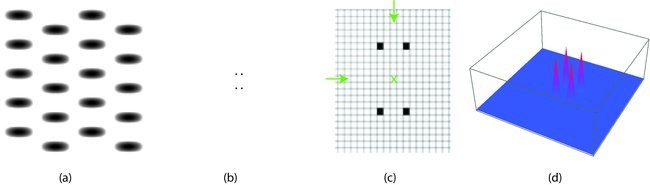2
(a) A (5,2) region from the sinusoidal model. (b) The Fourier transform of (a), called the spectrum, consists of four black dots located near the center. (c) A zoomed-in version of the spectrum (b) showing the location of the black pixels corresponding to the frequencies of the oscillations. The ‘x’ in the center is added to make it easier to count +/-2 pixels in the horizontal direction and +/-5 pixels in the vertical direction. (d) The same spectrum is plotted in a (pseudo) 3D landscape where the four ‘black dots’ are viewed as four red peaks.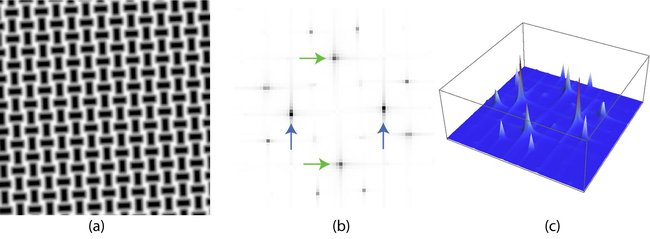3
A portion of the simplified rectangular model (a) and its magnitude spectrum (b)-(c). The periodicity of the vertical and horizontal patterns are visible in the spectrum as pairs of dark dots. The distance from the center/origin to these vertical and horizontal pairs is proportional to the frequency of the stripes in the two directions. In the 3D view, the periodicities of the vertical and horizontal patterns are specified by the locations of the peaks in the landscape.

What do the spectra look like when Fourier analysis is applied to canvas X-rays instead of to simplified artificial images such as figure 3(a)? Figure  shows three typical spectra drawn from various canvas X-rays. As in the sinusoidal and rectangular models, the distance in pixels from the origin (the middle) corresponds to the number of oscillations between dark and light across the region, and hence is proportional to the frequencies and to the thread counts in threads/cm. Therefore, in the majority of cases, the spectrum is a reasonable surrogate for the number of threads in the region, that is, the Fourier frequencies are proportional to the ‘thread count’ in the canvas X-ray.

There are several advantages to the Fourier approach. First, the detailed shape of the signal is not particularly important; the best match will typically occur at the correct frequency irrespective of the exact contour of the waves, that is, irrespective of the exact shape and placement of the threads. This is illustrated in figures 2 – 4, where similar qualitative patterns of peaks arise from regions with quite different thread contours. In essence, the Fourier transform shows the repetitive pattern, not the details of the pattern itself. Second, the spectrum tends to be robust to noise and outliers; adding even large amounts of digital noise does not change the answer. This is illustrated in figure  for the simplified sinusoidal pattern.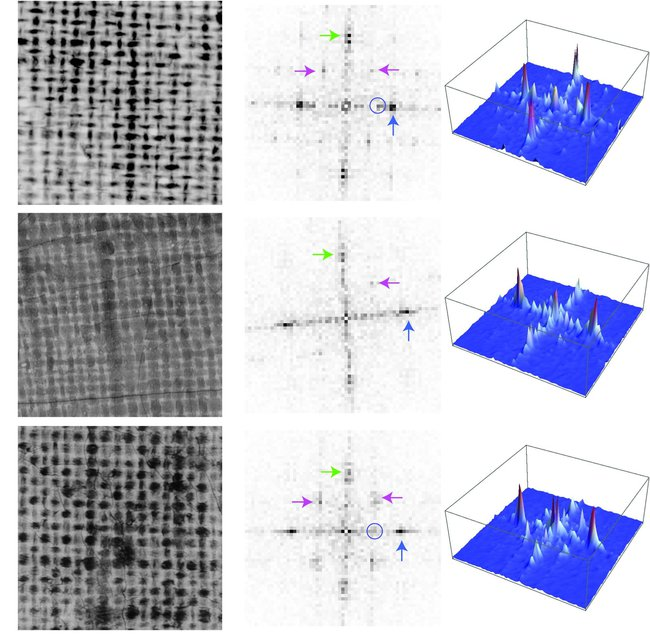4
Three regions of canvas X-rays and their spectra show many typical features. Dark spots in the spectra near the vertical axes (as indicated by the green arrows) represent the frequency of the horizontal threads; dark spots in the spectra along/near the horizontal axes (as indicated by the blue arrows) represent the frequency of the vertical threads. The blobs near the 45 and 135 degree lines (pointed to by the purple arrows) can also be used in the estimation of the frequencies. The small blue circles on the horizontal axes encircle local peaks, which are discussed later.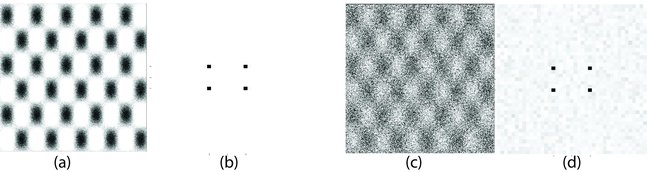5
The clean pattern on the left (a) has a Fourier transform consisting of four black dots (b). The noisy version on the right (c) has a Fourier transform (d) that is qualitatively similar, though a close look reveals many randomly located greyish areas in the background.

A third advantage is that even if a cycle or two is missing from the data (say due to over- or undersaturation in the X-ray), the correct frequency can still be reported. This is illustrated in figure , which shows a tile and its spectrum in the top images. The tile is then edited to remove portions by drawing in black (to mimic underexposure) and other portions by drawing in white (to mimic overexposure). The location of the peaks in the resulting spectrum are essentially unchanged, and the estimation of the frequencies in the horizontal and vertical directions are the same, despite the missing portions of the image.

On the other hand, there are also disadvantages to the Fourier approach. Perhaps the most important limitation is that the count needs to be (roughly) uniform over the region. Imagine a region in an X-ray which starts at the left hand side with a large thread count, and changes gradually to a small thread count at the right hand side. The Fourier transform will balance the large and the small counts, and report an average of the thread counts (or more properly, an average of the frequencies). This means that the count in each region should be relatively constant. For Vermeer’s canvases, there is often significant variation in thread density over 1 cm2 regions, and so the regions must be chosen smaller than this. Empirically, squares with sides 0.5 cm seem to be a good compromise, and this is the size of the regions in the X-rays used in chapter 6. Canvases of different eras or of different quality may benefit from counting larger or smaller regions.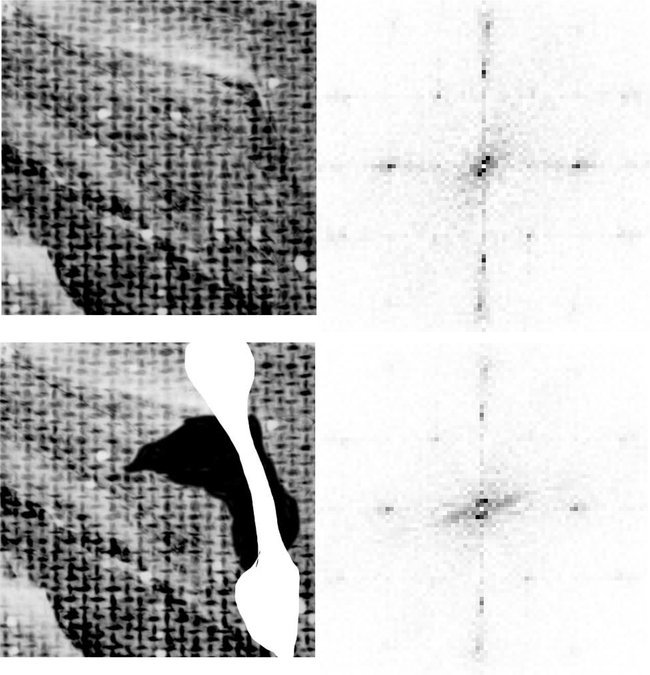6
The Fourier transform can often return accurate estimates of the frequencies even when part of the image is missing. The top images show an X-ray tile and its spectrum. The tile is then masked in both black and white (to simulate regions of dropout and overexposure), and its spectrum is taken again. While not identical, important parts of the spectra such as the locations of the peaks near the axes, are quite similar.